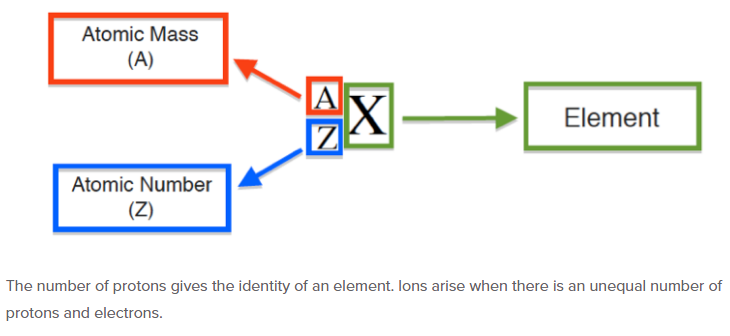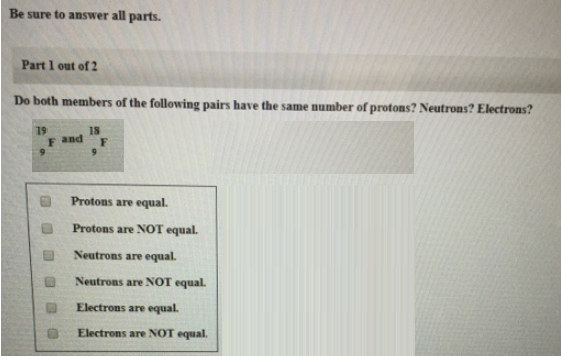# Problem: Be sure to answer all parts.Part 1 of 2Do both members of the following pairs have the same number of protons? Neutrons? Electrons? 19F9 and 18F9            (i) Protons are equal. (ii) Protons are NOT equal. (iii) Neutrons are equal. (iv) Neutrons are NOT equal. (v) Electrons are equal. (vi) Electrons are NOT equal.

###### FREE Expert Solution(i) Protons are equal. (True, atomic number = proton)

84% (145 ratings)###### Problem Details

Be sure to answer all parts.

Part 1 of 2

Do both members of the following pairs have the same number of protons? Neutrons? Electrons?

19F9 and 18F9

(i) Protons are equal.

(ii) Protons are NOT equal.

(iii) Neutrons are equal.

(iv) Neutrons are NOT equal.

(v) Electrons are equal.

(vi) Electrons are NOT equal.Frequently Asked Questions

What scientific concept do you need to know in order to solve this problem?

Our tutors have indicated that to solve this problem you will need to apply the Isotopes concept. You can view video lessons to learn Isotopes. Or if you need more Isotopes practice, you can also practice Isotopes practice problems.